# Realized volatility

The realized volatility is a new rising concept in the financial literature. It is derived from the realized variance and introduced by Bandorff-Nielssen and Sheppard. It is often used to measure the price variability of intraday returns. Although it can also be used at lower data frequencies.

## Realized volatility formula

In order to calculate it, you first need to calculate the log returns of the security as shown in the formula below.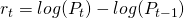In a next step, the realized volatility is calculated by taking the sum over the past N squared return.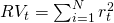The realized volatility is simply the square root of the realized variance.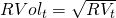## Annualizing realized volatility

When having calculated the realized variance of a single day, this can be annualized in the following way. By calculating the realized variance of a single day using high frequency data, the annualized realized variance equals the daily realized variance multiplied by the amount of trading days.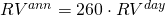The annualized realized volatility is simply the square root of the realized variance.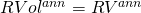## Summary

The realized volatility, or in general the realized variance, is a new financial volatility concept unknown to many investors. It is a popular technical to measure intraday price risk. As such it is useful to extend standard risk management practices with this approach.

### Return calculation

Want to have an implementation in Excel? Download the Excel file: realized volatility